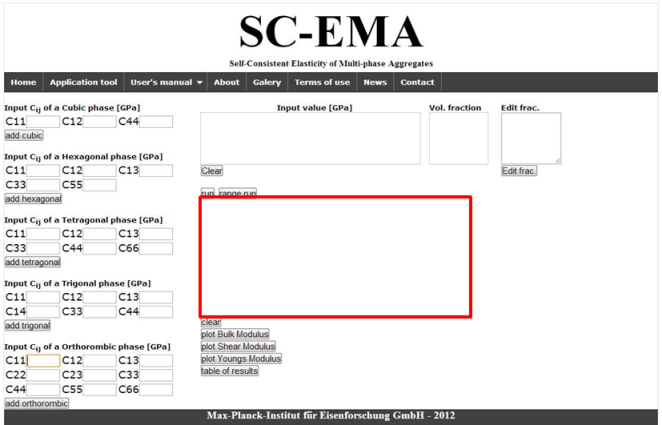SC-EMA

Self-Consistent Calculations - Elasticity of Multi-phase Aggregates

#### Step by step usage instructions:

1. Choose the number of phases.
2.#### For 1-Phase:

3. Choose the crystal symmetry.
4.5. Put in elastic constants (GPa). The number of required elastic constants depends on the chosen crystal symmetry.
6.7. Clicking on the “Run” button starts the calculation of homogenized elastic moduli. The input elastic constants can be visualized in the form of a directional dependence of the single-crystalline Young’s modulus by using the “plot youngs modulus” button.
8.9. Result window showing the value of homogenized polycrystalline elastic moduli calculated for your input parameters.
10.11. Clear the result from result window.
12.#### For 2-Phases & 3-Phases:

13. Please put in single-crystalline elastic constants for each phase present in your composite and then use the “add *” button (with * standing for a specific symmetry class).
14.15. The chosen elastic constant and crystal type will show in this window.
16.17. Clear button, for clearing the elastic constant input data.
18.19. The volumetric fractions of different phases present in your composite appear in this window. This automatic procedure sets volumetric fraction for each phase for you. The sum of all volumetric fractions must be equal to 1.
20.21. For editing the volumetric fraction of phases, after typing in the new values, please push the button “update conc.” 2 times, until it appears in the concentration window.
22.23. Result window, where the result will show in this window.
24.25. (Range) Run & Run the calculation. The “Run” button is for calculating homogenized polycrystalline elastic moduli for a specific set of volumetric fractions of phases in 2/3-phase composites. In contrast, The "Range Run" button is for calculating homogenized moduli for a set of volumetric fractions (from 0 - 1 for each phase). The resulting moduli are then visualized in graphs as function of volumetric fractions of phases. Please mind the fact that this option makes calculations 10 (70) times longer in case of 2 (3) phase composites as compared with a single run for one specific set of volumetric fractions of phases.
26.27. Clear the result which is showing in the result window.
28.29. Visualization of graphs with moduli for 2-phase or 3-phase composites. Please mind the fact that these graphs appear as pop-ups and so you need to have “Allow pop-ups” option in your browser activated. Alternatively to graphs, the "Table of result" button provides you with the result in a form of a text table (again showing up as a pop-up).
30.